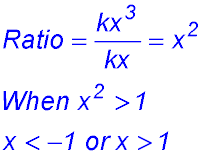Dr. J's Maths.com
Where the techniques of Maths
are explained in simple terms.

Sequences & Series - Geometric - the General term.
Test Yourself 1.

 Finding a term 1. Tn = 3×2n. T5 = 3 × 25 = 96. 2. T1 = 2 and r = 5 T6 = 2 × 55 = 6,250 3. T1 = -5 and r = -4 T8 = -5 × (-4)7= 81,920 4. T1 = 3 and T4 = 192 ∴ 192 = 3× r3 r3 = 64 r = 4 First four terms are 3, 12, 48 and 192. Finding a 1st term 5. T3 = 18 and r = 3 18 = T1 × 32 T1 = 2 6.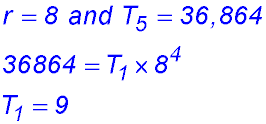Finding a difference 7.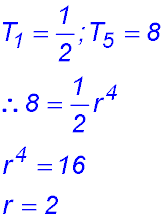8.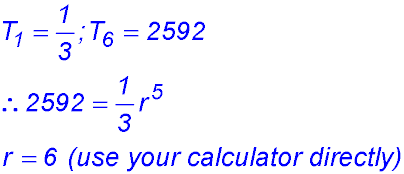Finding the number of terms 9.10.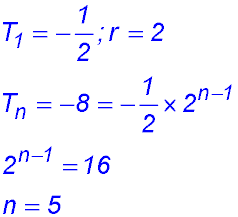Given 2 terms 11.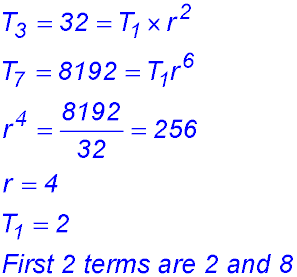12.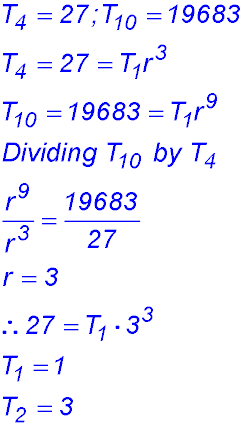13.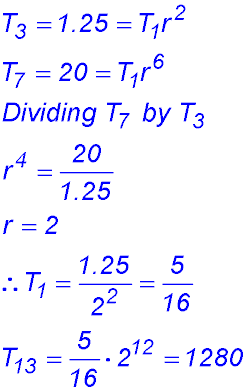14.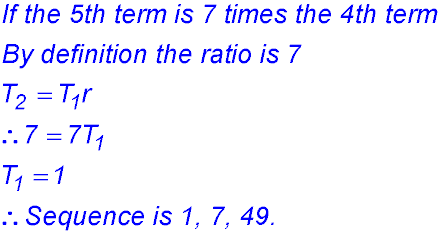Miscellaneous 15.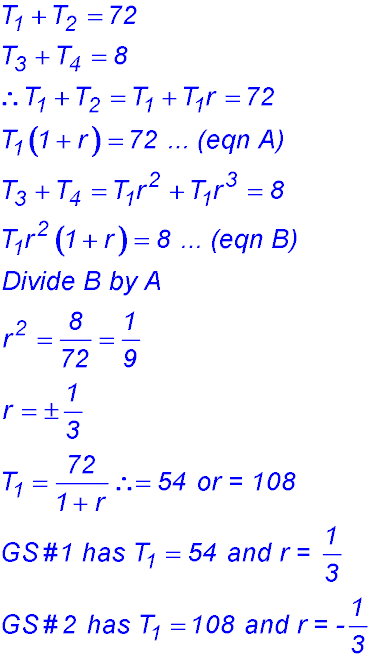16.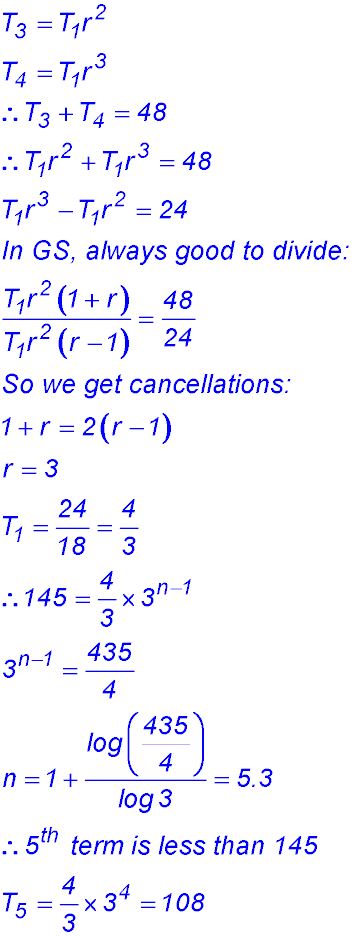17. The three terms are in geometric progression and so they have the same ratio between them. The ratio can be calculated in 2 different ways - by ratioing the 2nd term to the 1st term and then the 3rd term to the 2nd term. As these are the same, equate them.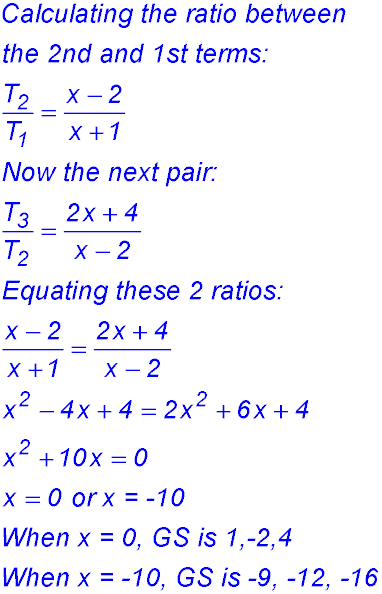18. Same approach as for the previous question. Calculate the common ratio in two different ways and equate the results.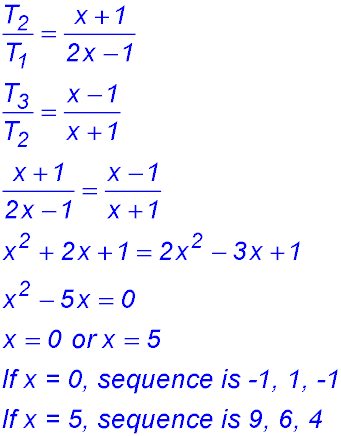19.20.